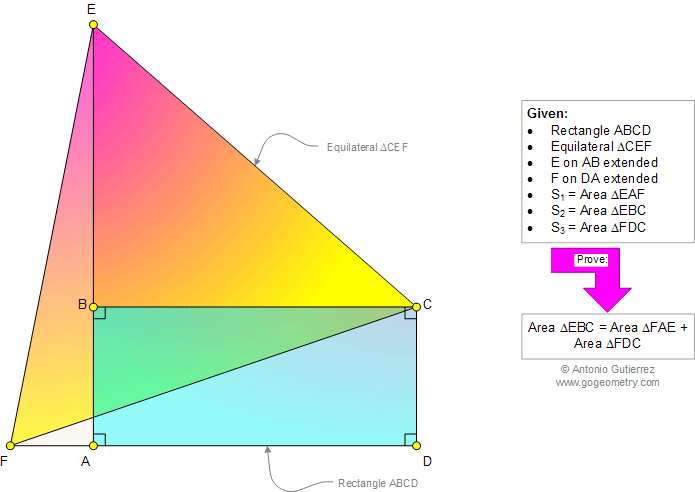# Geometry Problem 971: Equilateral Triangle, Rectangle, Common Vertex, Sum of Right Triangles Areas. Level: School, College, Mathematics Education

< PREVIOUS PROBLEM  |  NEXT PROBLEM >

 The figure shows a rectangle ABCD and equilateral triangle CEF (E on AB extended, F on DA extended). Prove that: Area triangle EBC = Area triangle FAE + Area triangle FDC.Home | Search | Geometry | Problems | All Problems | Open Problems | Visual Index | 10 Problems | Problems Art Gallery |  Art | 971-980 | Triangles | Equilateral Triangle | Rectangle | Areas | Right Triangle Area | by Antonio Gutierrez